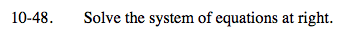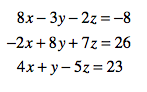### Home > A2C > Chapter 10 > Lesson 10.1.2 > Problem10-48

10-48.

Solve the system of equations below. Homework Help ✎ 8x − 3y 2z = −8
−2x + 8y + 7z = 26
4x + y − 5z = 238x − 3y − 2z = −8 →

4(−2x + 8y + 7z = 26) →

2(−2x + 8y + 7z = 26) →

4x + y − 5z = 23 →

8x − 3y − 2z = −8

−8x + 32y + 28z = 104

29y + 26z = 96 (a)

−4x + 16 + 14z = 52

4x + y − 5z = 23

17y + 9z = 75 (b)

Now use (a) and (b) to find y and z, and then find x.

$\left(\frac{1}{2},\,6,\,-3\right)$